# Colleges with the highest SAT scores in Connecticut

Top 10 colleges in Connecticut with the highest SAT scores
Looking for the colleges with the highest SAT scores in Connecticut? Well you're in luck! We've compiled a national college database and have created a list of the top 10 universities with the highest SAT scores in Connecticut. These are the schools whose applicants had the highest average SAT scores in Connecticut. And, since these tests are meant to determine academic prowess, they are arguably the schools with the most academically proficient students. You could even say these are the best colleges in Connecticut. We also include each college's ACT scores and acceptance rate so that you can see where you would have the easiest or hardest time getting in. Read on to find out more.

## Yale University SAT scores

The average SAT score for Yale University is 1515.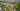The average SAT score of 1515 breaks down into:

• SAT math: 770

The average ACT score for Yale University is 34 and their acceptance rate is 6.1%.

## Wesleyan University SAT scores

The average SAT score for Wesleyan University is 1395.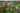The average SAT score of 1395 breaks down into:

• SAT math: 700

The average ACT score for Wesleyan University is 32 and their acceptance rate is 16.3%.

## Trinity College SAT scores

The average SAT score for Trinity College is 1380.The average SAT score of 1380 breaks down into:

• SAT math: 710

The average ACT score for Trinity College is 31 and their acceptance rate is 33.5%.

## Connecticut College SAT scores

The average SAT score for Connecticut College is 1358.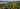The average SAT score of 1358 breaks down into:

• SAT math: 680

The average ACT score for Connecticut College is 30 and their acceptance rate is 37.8%.

## University of Connecticut SAT scores

The average SAT score for University of Connecticut is 1315.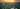The average SAT score of 1315 breaks down into:

• SAT math: 670

The average ACT score for University of Connecticut is 29 and their acceptance rate is 48.8%.

## Fairfield University SAT scores

The average SAT score for Fairfield University is 1270.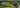The average SAT score of 1270 breaks down into:

• SAT math: 640

The average ACT score for Fairfield University is 28 and their acceptance rate is 60.3%.

## Sacred Heart University SAT scores

The average SAT score for Sacred Heart University is 1175.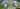The average SAT score of 1175 breaks down into:

• SAT math: 590

The average ACT score for Sacred Heart University is 25 and their acceptance rate is 60.5%.

## Eastern Connecticut State University SAT scores

The average SAT score for Eastern Connecticut State University is 1140.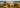The average SAT score of 1140 breaks down into:

• SAT math: 560

The average ACT score for Eastern Connecticut State University is 23 and their acceptance rate is 63.6%.

## Quinnipiac University SAT scores

The average SAT score for Quinnipiac University is 1135.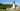The average SAT score of 1135 breaks down into:

• SAT math: 560

The average ACT score for Quinnipiac University is 24 and their acceptance rate is 78.5%.

## University of New Haven SAT scores

The average SAT score for University of New Haven is 1131.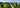The average SAT score of 1131 breaks down into:

• SAT math: 560